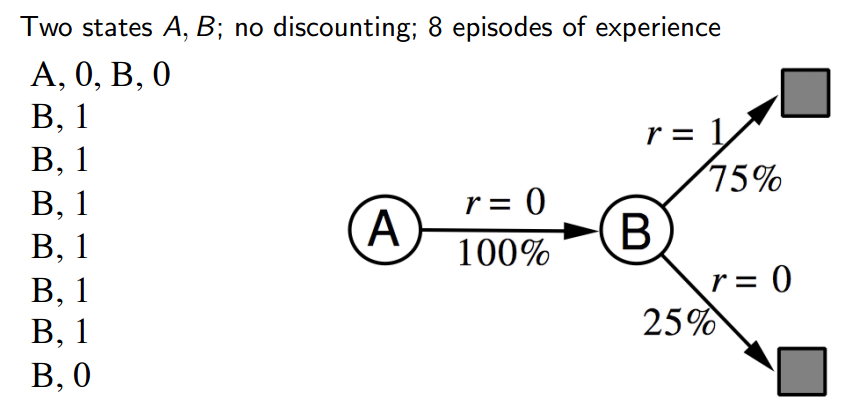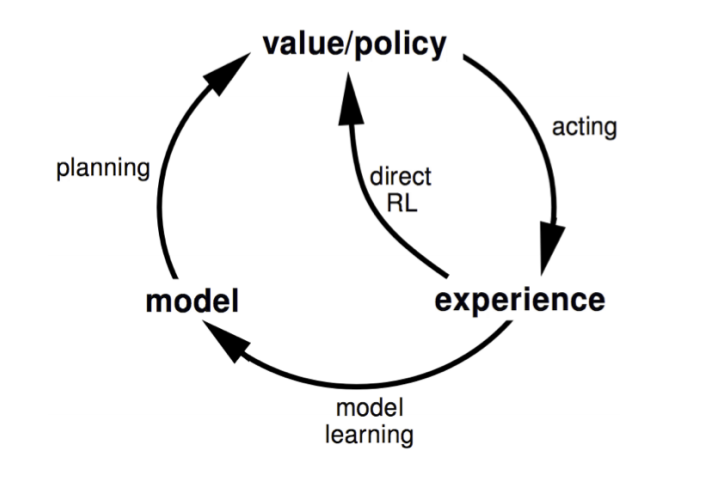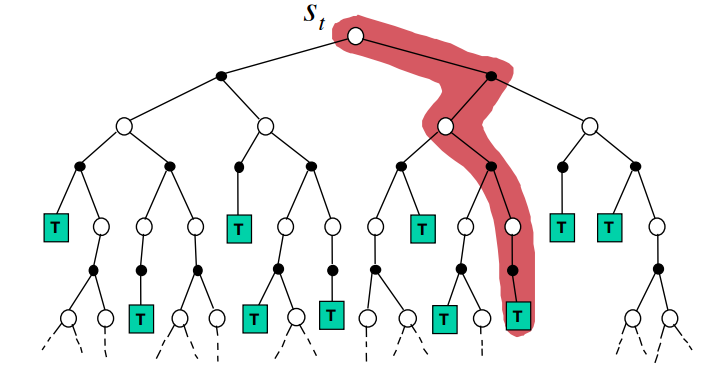【转载请注明出处】chenrudan.github.io#### 1.内容回顾

environment的参数化好处在于，避免了状态多policy复杂难学，它像在模拟出游戏的规则，这样就能通过搜索树的方式获得value function，并且能够通过监督学习方式来学习model。例如输入是状态$s$输出是状态$s’$，此处就能给一定的监督信息，说明$s’$取什么样的值比较好。而缺点在于用一个近似的model来构造一个近似的value function，两个近似都会存在一定的误差。

#### 2.Model-Based Reinforcement Learning

model的形式可以表现为$S,A,P,R$，假设状态集和动作集是已知的，把状态转移和奖赏表示成状态和动作的独立概率分布为$P[S_{t+1}, R_{t+1}|S_t, A_t] = P[S_{t+1}|S_t, A_t]P[R_{t+1}|S_t,A_t]$。目标就是从一段执行序列中$S_1,A_1,R_2,…,S_T$来估计model，这样就能看成一个监督问题，把$S_t,A_t$当成模型输入，那么即$R_{t+1},S_{t+1}$就能看成模型的输出。学习$R_{t+1}$是一个回归问题，可以用最小均方差等方法来解决，而学习$S_{t+1}$则是一个密度估计问题，可以用KL divergence等方法来解决。#### 3.Integrated Architectures• 针对集合$S$和$A$所有值初始化$Q(s,a)$和$Model(s,a)$
• 循环
• ==选择当前状态为$S$，通过$\epsilon-$greedy选择一个$A$
• ==执行$A$，获得reward R和下一个state $S’$(前两步都来自real experience)
• ==由Q-Learning更新动作值函数$Q(S,A) = Q(S,A) + \alpha[R+\gamma max_aQ(S’,A)-Q(S,A)]$
• ==由获得的$R,S’$来学习模型$Model(S,A)$
• ==循环n次
• ====随机选择一个$S$和$A$
• ====基于模型得到$R,S’$
• ====同样的方法更新动作值函数Monte-Carlo Tree Search算法的流程如下

• 给定一个model $M_v$
• 从一个重要状态出发$s_t$在当前的simulation policy下模拟出K个episodes，即得到$( s_t, A_t^k, R_{t+1}^k, S_{t+1}^k…,S_T^k) _{k=1}^K~M_v,\pi$
• 根据episodes构造搜索树
• 由Monte-Carlo Learning每次访问到$s,a$都记录一次最后求所有episodes的平均得到$Q(s,a) = \frac{1}{N(s,a)} \sum_{k=1}^K\sum_{u=t}^T 1(S_u,A_u=s,a)G_u$
• 然后由最大值函数得到当前最佳的action，即$a_t = \underset{a\in A}{argmax}Q(s_t,a)$